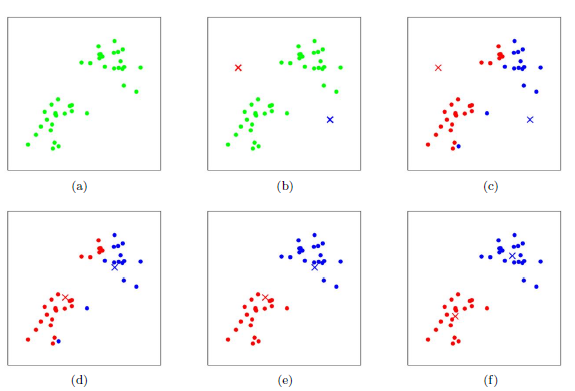# K-Means 算法基础

K-Means 算法基础

## 基本原理

K-Means 作为一种最简单的聚类算法，在数据挖掘中有着比较广泛的应用。如下图所示，给你一批数据（绿色的点），让你将数据划分成2类，这就是K-Means要解决的问题。1. 随机选取K个中心点 图（b）
2. 遍历所有数据，将每个数据划分到最近的中心点 图(c)(d)(e)
3. 计算每个聚类的平均值，并作为新的中心点
4. 重复上述过程，直到这k个中线点不再变化（收敛了），或执行了足够多的迭代 图(f)

## 距离的种类

### 欧氏距离

$$d = \sqrt{(x-y)^2} = \sqrt{\sum_{i=1}^m (x_i -y_i)^2}$$

### 曼哈顿距离

$$d = \sum_{i=1}^{m} |x_i -y_i|$$

### 闵可夫斯基距离

$$d = (\sum_{i=1}^{m} (x_i -y_i)^{\lambda}) ^{\frac{1}{\lambda}}$$

## K-MEANS的缺点

K-Means主要有两个最重大的缺点，都和初始值有关：

1. 第一个问题是初始聚类中心的选择问题，由于初始的聚类中心是完全随机的并且不同的聚类中心会带来完全不同的聚类结果，因此这个因素大大影响着K-MEANS的效果。
2. 聚类数量K要求事先给定。在大部分情况下，由于数据维度很高，这个K值的选定是非常难以估计的。很多时候，事先并不知道给定的数据集应该分成多少个类别才最合适。

## 初始聚类中心

### 多次运行

$$SSE = \sum_{i=1}^K \sum_{x \in \omega_i} |x-u_i|^2$$

$$u_i = \frac{1}{N_i} \sum_{x \in \omega_i} x$$

### K-MEANS++算法

1. 从输入的数据点集合中随机选择一个点作为第一个聚类中心
2. 对于数据集中的每一个点x，计算它与最近聚类中心(指已选择的聚类中心)的距离D(x)之和
3. 选择一个新的数据点作为新的聚类中心，选择的原则是：D(x)较大的点，被选取作为聚类中心的概率较大
4. 重复2和3直到k个聚类中心被选出来
5. 利用这k个初始的聚类中心来运行标准的k-means算法

## 聚类数量K的选取

1. 对于第$i$个元素$x_i$，计算$x_i$与其同一个簇内的所有其他元素距离的平均值，记作$a_i$，用于量化簇内的凝聚度。$a_i = \frac{1}{N}\sum_{j=1}^{N}d(x_i,x_j)$
2. 选取$x_i$外的一个簇$b$，计算$x_i$与b中所有点的平均距离，遍历所有其他簇，找到最近的这个平均距离,记作$b_i$，用于量化簇之间分离度。$b_i = \min\limits_{b} { \frac{1}{N_b}\sum_{j=1}^{N_b}d(x_i,x_j)}, x_j \in \omega_b$, 其中$N_b$表示类别b中的元素数量
3. 对于元素$x_i$，轮廓系数 $s_i = (b_i – a_i)/max(a_i,b_i)$
4. 计算所有x的轮廓系数，求出平均值即为当前聚类的整体轮廓系数

$$SC=\frac{1}{N} \sum_{i=1}^{c} \sum_{j=1}^{N_i} s_j$$

Please Indicate the Source When Reproduce